# Mental Addition of 2-Digit Numbers• To add 2-digit numbers mentally, it is important to know the addition results shown above.• Add the tens digits and then look at the ones digits to decide what the answer ends in.
• For example in 32 + 29, we add the tens digits first.
• 3 + 2 = 5.
• Adding the ones, 2 + 9 = 11.
• Because 11 ends in a 1, we know our answer ends in a 1.
• We increase the 5 in the tens column to a 6.
• 32 + 29 = 61.

Add the tens column and then the ones column separately.

The answer ends in the same digit that the sum of the units column ends in.• We first add the tens column digits.
• 3 + 4 = 7. We hold this number in our head.
• We now add the digits in the ones column.
• 6 + 9 = 15.
• So we add one to the tens column and our answer ends in a 5.
• The tens column increases from 7 to 8 and the ones column is 5.
• 36 + 49 = 85.Supporting Lessons#### Mental Addition of 2-Digit Numbers: Interactive Questions

There are various strategies for mental addition, however, with each method, it is important to hold values in your head. The fewer the steps, the better the method as it is easier to hold the numbers in your head.

## How to do Mental Addition

To do mental addition, use the following steps:

1. Add the digits in the tens column together and remember this result.
2. Add the digits in the units column together.
3. If this result is larger than ten then add one to your tens column answer from before.
4. The units column of the units column result is the units digit of your answer.

We only hold the result of the tens column in our head while we add the units column.

To add 2-digit numbers, it is first worth knowing the following 1-digit additions:In this first example, we have 32 + 29.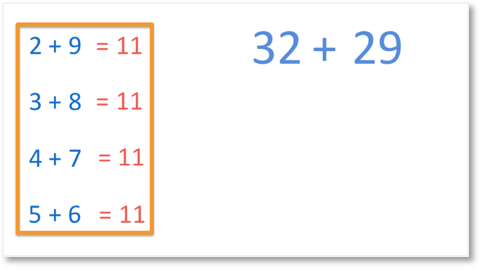The first step is to add the digits in the tens column.

3 + 2 = 5.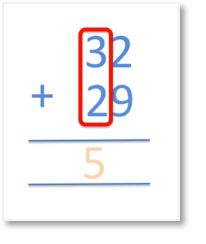The next step is to add the digits in the units column.

2 + 9 = 11

11 is larger than 10 and so, we need to add 1 to our result from adding the tens column. This is because adding 11 is the same as adding ten and then adding 1.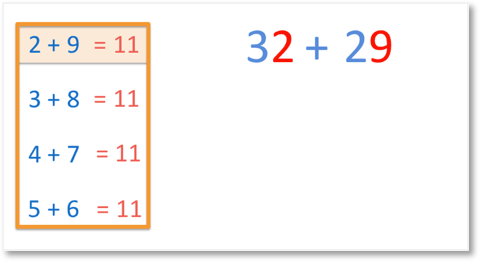The 5 in the tens column becomes a 6.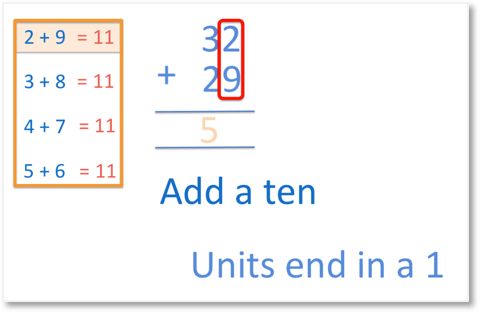Because 11 ends in a 1, we know that our digit in the units column of our answer is also a 1.32 + 29 = 61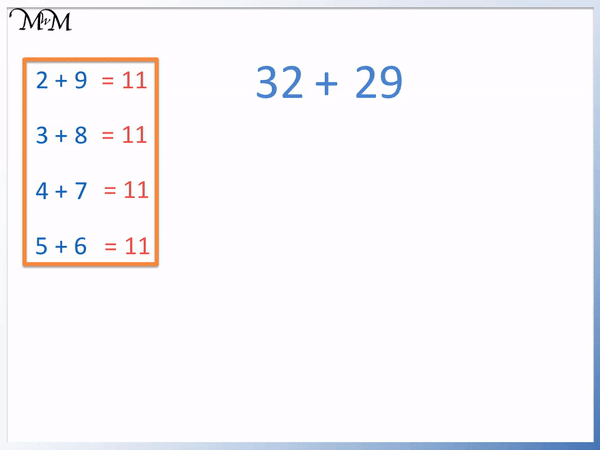In this next example we have the mental addition of the 2-digit numbers 44 + 28.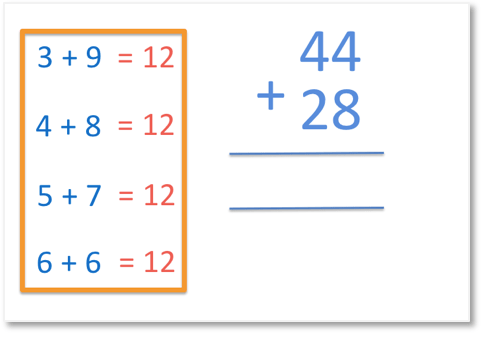The first step to adding two 2-digit numbers mentally is to add the digits in the tens column.

4 + 2 = 6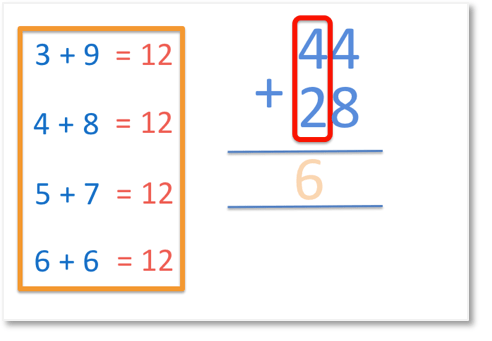The next step is to add the digits in the units column.

4 + 8 = 12

This means that we increase the tens column by 1 and our answer ends in a 2.The 6 increases to a 7.

The answer ends in a 2 because 12 ends in a 2.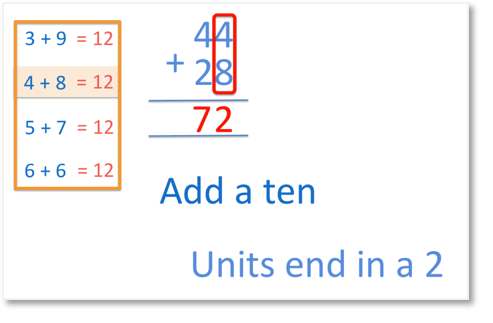44 + 28 = 72

When teaching mental addition, we recommend trying to hold the first result in your head whilst carrying out the next part of the calculation. When first learning mental addition, start by writing the result a few times until you get the hang of the method.

Once you get better at performing the written method you will find that it is easier to remember the steps and it will be easier to hold numbers in your head.In this example of mental addition we have 28 + 25.

We will try and speed up our method.

2 + 2 = 4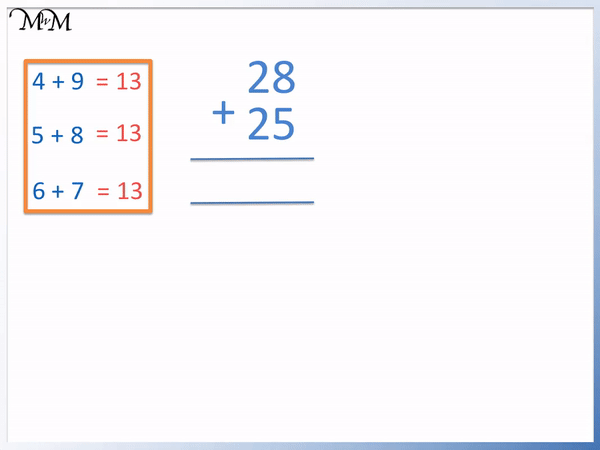8 + 5 = 13 and so, we increase 4 to 5 in the tens column. Our answer ends in 3.

28 + 25 = 53

Here is another example of adding 2-digit numbers mentally.

We have 36 + 49.

Adding the tens digits we have 3 + 4 = 7.

Adding the units digits we have 6 + 9 = 15.

We increase the 7 to an 8 and our answer must end in a 5.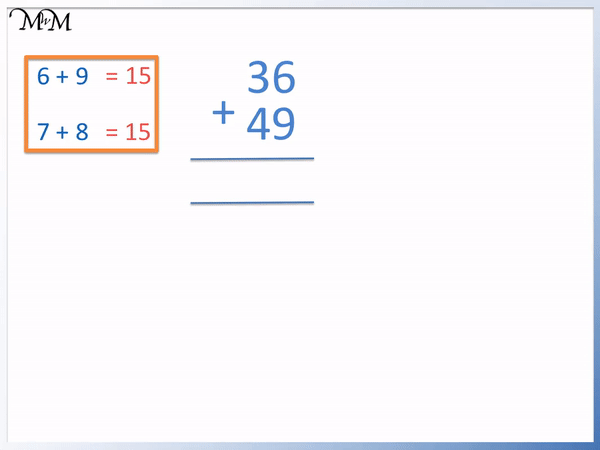36 + 49 = 85

In this example of adding mentally, we have 69 + 29.

Adding the tens, 6 + 2 = 8.

Adding the units, 9 + 9 = 18.

Therefore, we increase the 8 in the tens column to a 9 and our answer ends in an 8.69 + 29 = 98

With practice, this method is one of the quickest ways to add 2-digit numbers mentally.

When teaching mental addition, if your child struggles with the process, it is worth focusing on writing the steps down until they are mastered. This will make it easier to transfer the process to holding numbers in their head.Now try our lesson on 2-Digit Column Addition where we learn how to use the column addition method.error: Content is protected !!